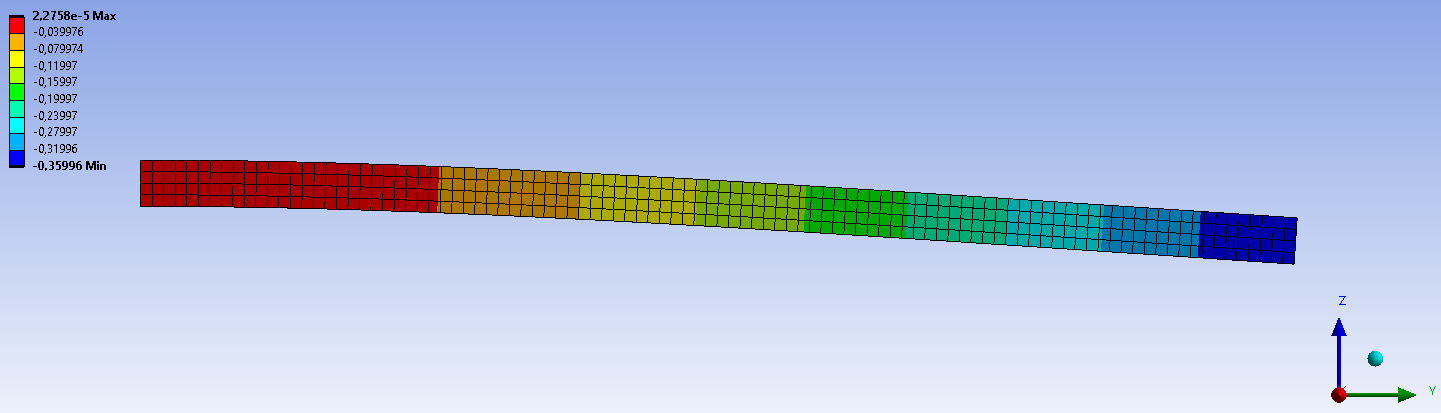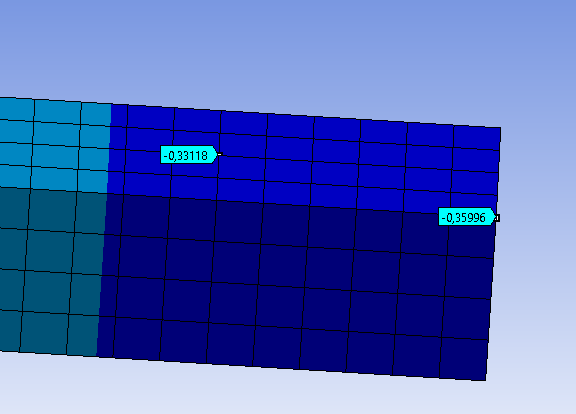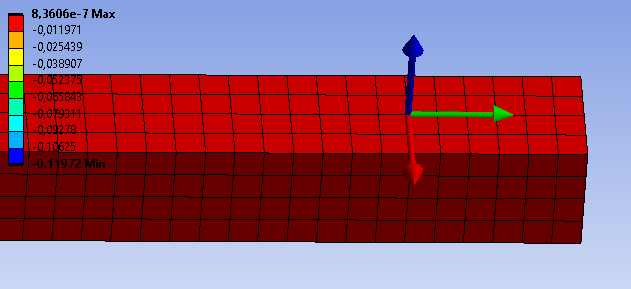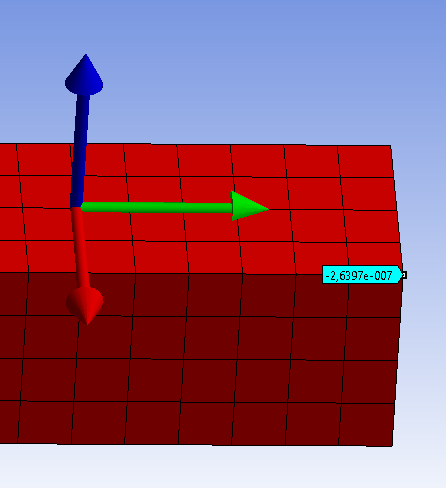IñigoTek
Subscriber
Hello,nThank you for the answer. I have downloaded the ACT tool and I have used it with an example to see how it works.nnMy example is a fixed beam under its own weight. The total deformation result in Z (exagerated) is what I am showing in the next image:nIn this result i have taken two nodes to see the distance between them. Compaing the Z coordinate of both nodes the distance ( in Z) is 0,03 mm.nTo prove the relative displacement tool, i have fix the coordinate system in on of the nodes and evaluate the result of relative displacement in Z.nNow I use the probe button to see the distance between te origin of the coordinate system (Node 1) and the other node which should be 0,03 mm as i calculated with total deformation. But my result is -2,63*10^(-7) mm which is wrong.nCan yo help me? I am not using well the tool?.Thank you, nIñigo.n